Skip to main content

# Main Classification Algorithms - Part 2Welcome to the second article on the main classification algorithms. Previously, we had explored the various classification measures, based on the evaluation metrics, as well as the first three classification algorithms: K-nearest neighbors (KNN), Decision Trees and Random Forests.

Classification is such an important and useful supervised machine learning tool that there are two other important algorithms worth mentioning: Logistic Regression and Naïve Bayes’ algorithm. We will be focusing on these below.

2. a. Logistic regression

Logistic regression is a linear classification technique that is used to predict the probability of a target value and to establish a linear relationship between the input and the output variables. Most commonly, the dependent variable is binary and thus has data coded as either 1, which stand for success/yes, or 0, which stands for failure/no). However, there can also be more than two categories of target variables, which therefore implies the use of a multinomial logistic regression.

i) Binary or binomial logistic regression

Binary, or binomial, logistic regression constitutes the simplest and most common form of logistic regression. It involves a dependent variable of only two possible types: either 1 or 0.

Since logistic regression is a linear model, the hypothesis function can be given as: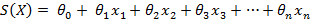If we set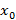=1, then the above formula is equivalent to: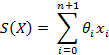​​​​​​​

In which case, X corresponds to the vector of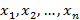Similarly, if we let ϑ  be the vector containing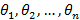, then we can rewrite the above formula as: S (X) = ϑX.

This hypothesis function is called the score function. Ideally, we wish to findsuch that:

• S(X)>0 when the dependent variable is 1
• S(X)<0 when the dependent variable is 0

Now, using the score function, we can compute the sigmoid function, used for the logistic regression models. This function results in values between 0 and 1 and this result can be interpreted as the probability of the observation X being of output variable 1. When applying this sigmoid function on our score function, we obtain our hypothesis function for our logistic regression, as follows: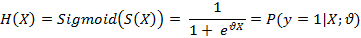Thus, this corresponds to the probability of X being of class 1 with the parameters ϑ . Equivalently, the probability of X being of class 0 is given by: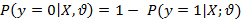The graph below represents the previous sigmoid function: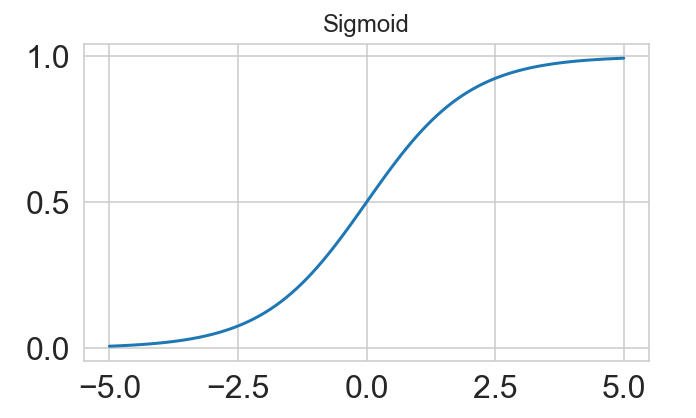ii) Multinomial logistic regression

In this kind of classification, the dependent variable can take 3 or more possible values or types. For instance, we could have a dependent variable of “Type A”, “Type B”, or “Type C”. The dependent variable would have to be of unordered type, meaning that the types would have no quantitative significance. The above formulas presented in the binary logistic regression are adaptable for the multinomial version.

2. b. Naïve Bayes

Naïve Bayes algorithms is a supervised classification machine learning technique, which relies on Bayes’ theorem and the assumption that all the dependent variables are independent of one another. In other words, the assumption implies that the presence of a variable in one class is independent to the presence of another variable in that same class. Therefore, any modification to one of the features should not directly impact the value of any other feature of the model.

Naïve Bayes classifiers require training data, like any supervised model, to estimate the parameters required for the classification. The interest of using is method is finding the probability of a label, or a class, given a set of features. We call this the posterior probability and can be calculated given the following formula: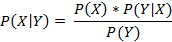​​​​​​​

where :

• Y is the set of features and X is the set of observations
• P(X|Y) is the posterior probability of a class and is given by: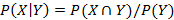​​​​​​​

• P(X) is the prior probability of the class
• P(Y│X) is the likelihood i.e. the probability of a predictor given the class and is given by: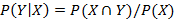• P(Y) is the prior probability of the dependent variable

Naïve Bayes models are usually used for the classification of documents, filtering spams, predictions and so on.

There exists numerous variations of the Naïve Bayes algorithm including Gaussian Naïve Bayes, Bernoulli Naïve Bayes and Multinomial Naïve Bayes. Below we will try to understand the Gaussian Naïve Bayes in a bit more detail.

i) Gaussian Naïve Bayes

Gaussian Naïve Bayes is an extension of Naïve Bayes, with the specificity that X follows a particular distribution known as the Gaussian or normal distribution. Hence, we can use the probability density function of this distribution to calculate the probability of likelihoods. To do so, we need to know the mean and variance of X and use the following formula: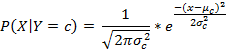​​​​​​​​​​​​​​

where:

• σ represents the variance of X and μ represents the mean of X
• c  represents a class of Y

### What’s next:​​​​​​​

Hopefully, the two previous articles will have given you a good understanding of the five main classification algorithms.

With all of the above information in mind, we can understand that various applications require different algorithms to be used, depending on the criteria of the problem at hand.

At Linedata, we have been incorporating some classification techniques into our products, such as to predict trade error scores. For more information about this use case, please view the upcoming article.